# Data - How to create bulk test/dummy records with random values

When building apps, it's useful to be able to populate tables with dummy or test records so that we can test and see how our app behaves. This post demonstrates formula to generate multiple records with random values, and how to add those records to a table.

When we build an app from an empty table, it's useful to populate the table with dummy data so that we can properly test the app. This includes checking how gallery controls appear when populated with data, whether search and edit features function correctly, and whether any part of our app doesn't return the expected data due to delegation limitations.

In this post, we'll walk through how to create formula to populate a Dataverse table.

## Example - the table where we'll create test records

The screenshot beneath illustrates the table that we'll use, which is called 'Property'. The data source is Dataverse for Teams database, but the technqiue in this post equally applies to SharePoint, Excel, or any other supported data source.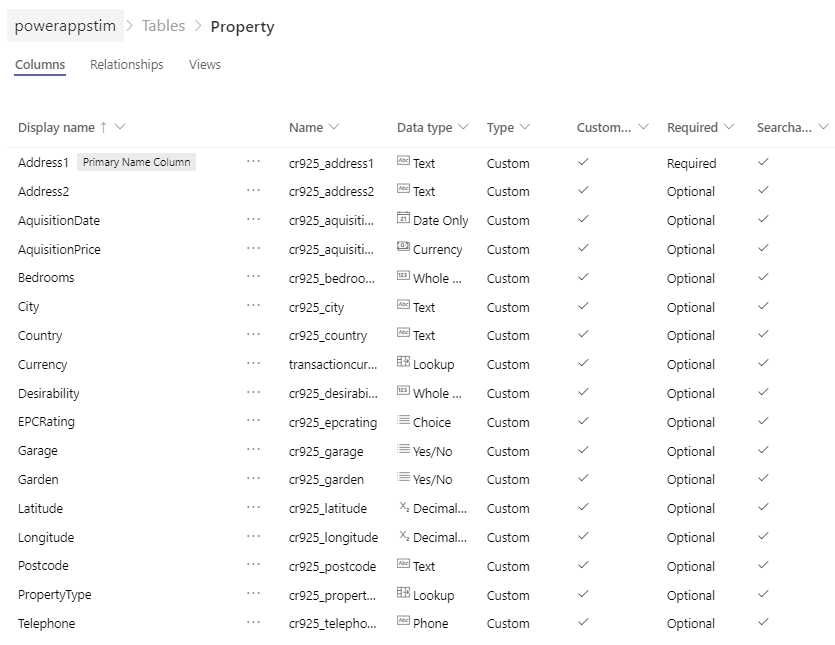The property table includes a range of columns of various data types including text, dates, numbers, yes/no columns, and a LookUp column that references a table called 'Property types'.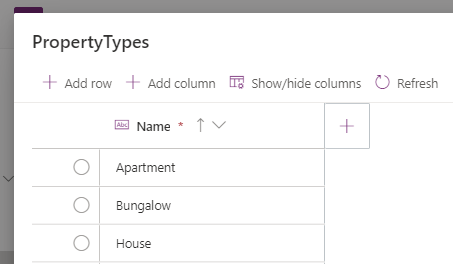## Basic Syntax - How to add 100 dummy/test records to a table

Let's look at the basic syntax to create 100 test records. From our app, we connect to the source table, and apply the following formula to a button

`Collect(Properties,         ForAll(Sequence(100,1,1),               {Address1:"Address " & Text(Value)}         ))`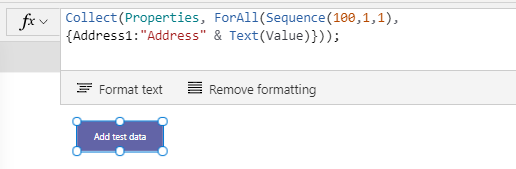This formula relies on several key functions. The Collect function adds multiple new records to a table (in this example, Properties is the name of the table)

The Sequence function returns a sequence of records. This function takes three arguments - the number of records to generate, the start value, and the 'step' value (ie, the incremental step/value between each record in the sequence).

For each record in this sequence, we apply the ForAll function to return a record with the field name "Address1", with a value that matches the number of the sequence and is prefixed with the text "Address".

To highlight the output of this formula, here's a screenshot of the data view of the table.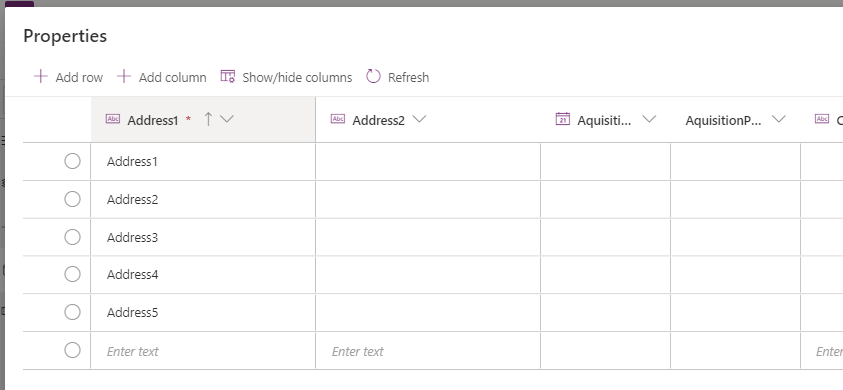Now that we understand the basic syntax to create a set of records, we'll extend our formula to populate the other fields.

But before we do this, let's cover the syntax to generate random values. This will enable us to populate the fields in our table (such as aquistion date, number of bedrooms.property type) with random values.

## Generating random whole numbers

Starting with how to generate random numbers, here's the formula to create a random number between a start and end value (1 and 8 in this example).

`With({randMin:1, randMax:8},      RoundDown(Rand()*(randMax-randMin + 1) + randMin,0)     )`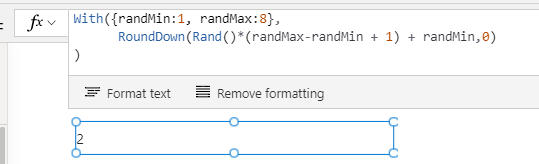This formula relies on the Rand function. The Rand function returns a pseudo-random number that's greater than or equal to 0, and less than 1.

The output from the Rand function contains multiple decimal places (eg, 0.78896559). Since this is always less than 1, we multiply this by the maximum number we want to generate, and we call the RoundDown function to remove the fractional units.

## Generating random decimal numbers

To generate random decimal numbers, we can modify our formula from above, and specify the target number of decimal places in the call to the RoundDown function.

As an example, here's the formula to generate a random value between 100,000 and 200,000, with 2 decimal places.

`With({randMin:100000, randMax:200000},      RoundDown(Rand()*(randMax-randMin + 1) + randMin,2)     )`

## Generating random dates

To generate a random date, we can generate a random number, and to add or subtract that number from a base date. Here's how to how to generate a random date within the past four years (eg - 365 days *4).

`With({randMin:1, randMax:(365 * 4)},      DateAdd(Now(),              -1 * RoundDown(Rand()*(randMax-randMin + 1) + randMin,0)      )     )`

##This formula calls the DateAdd function and subtracts the random number of days from todays date.

## Generating random LookUp values

Where we want set a choice column to a random record, we can call the Shuffle function. The Shuttle function re-orders the records in a table in a random way, and we can select the first record from the result to retrieve a random record.

`First(      Shuffle(PropertyTypes))`

## Generating random boolean, yes/no, true/false values

To generate a random boolean value, we can apply the formula beneath.

`Round(Rand(),0) = 1`This formula generates a random number and rounds it to either 0 or 1. We apply the equality (=) operator to return true if the value is 1, or false if it's 0.

## How to create test records with random data

At this stage, we can now put together all the formula from above, and to generate a set of records that include random values for the aquisition date, number of bedrooms, garden, and property type fields.

`Collect(Properties,         ForAll(Sequence(100,1,1),                With({                      roomsMin:1,                      roomsMax:8,                      daysMax:(365*5)                    },                    {                      Address1:"Address " & Text(Value),                      AquisitionDate:                         DateAdd(Now(),                                 -1 * RoundDown(Rand()* daysMax,0)                         ),                      Bedrooms:                         RoundDown(Rand()*(roomsMax-roomsMin + 1) + roomsMin,0),                      Garden: If((Round(Rand(),0) = 1),                                 'Garden (Properties)'.Yes,                                 'Garden (Properties)'.No                               ),                      PropertyType:                          First(Shuffle(PropertyTypes))                    }               )         ))`

A notable point here is the syntax that set's a Dataverse yes/no field. It's not syntactically correct to set the 'Garden' field to true or false and therefore, we must apply an if statement to return a Yes/No enumeration value.

The screenshot beneath shows the output of this formula highlights how we've successfully generated random values for a range of data types.## Conclusion

When building apps, it's very useful to be able to populate tables with dummy records. This post demonstrated the formula we can use to generate random values, and to add multiple records to a data source
•   Categories:
• data